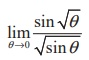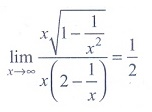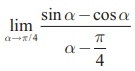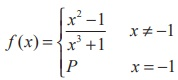Home | | Maths 11th std | Exercise 9.6: Choose the correct answer

# Exercise 9.6: Choose the correct answer

Multiple choice questions with answers / choose the correct answer with answers - Maths Book back 1 mark questions and answers with solution for Exercise Problems - Mathematics : Differential Calculus - Limits and Continuity

Differential Calculus - Limits and Continuity (Mathematics)

Choose the correct or the most suitable answer from the given four alternatives.

(1)(1) 1

(2) 0

(3) ∞

(4) −∞

Ans: (2)

Solution(2)(1) 2

(2) 1

(3) −2

(4) 0

Ans: (3)

Solution(3)(1) 0

(2) 1

(3) √2

(4) does not exist

Ans: (4)

(4)(1) 1

(2) - 1

(3) 0

(4) 2

Ans: (1)

Solution(5)is

(1) e4

(2) e2

(3) e3

(4) 1

Ans: (1)

(6)(1) 1

(2) 0

(3) - 1

(4) 1/2

Ans: (4)

Solution(7)(1) log ab

(2) log (a/b)

(3) log (a/b)

(4) a/b

Ans: (2)

(8)(1) 2 log 2

(2) 2(log 2)2

(3) log 2

(4) 3 log 2

Ans: (2)

(9) If, then the value of limx→0 f (x) is equal to

(1)  -1

(2) 0

(3) 2

(4) 4

Ans: (2)

(10)(1) 2

(2) 3

(3) does not exist

(4) 0

Ans: (3)

Solution(11) Let the function f be defined by f (x)=, thenAns: (4)

Solution(12) If f : R→ R  is defined byfor x R, then limx→3 f (x) is equal to

(1) - 2

(2) - 1

(3) 0

(4) 1

Ans: (3)

(13)is

(1) 1

(2) 2

(3) 3

(4) 0

Ans: (4)

Solution(14)  If, then the value of p is

(1) 6

(2) 9

(3) 12

(4) 4

Ans: (3)

(15)is

(1) √2

(2) 1/√2

(3) 1

(4) 2

Ans: (1)

(16)is

(1) 1/2

(2) 0

(3) 1

(4) ∞

Ans: (1)

Solution(17)(1) 1

(2) e

(3) 1/ e

(4) 0

Ans: (1)

(18)(1) 1

(2) e

(3) 1/ 2

(4)  0

Ans: (1)

(19) The value ofis

(1) 1

(2) - 1

(3) 0

(4) ∞

Ans: (4)

(20) The value of, where k is an integer is

(1) - 1

(2) 1

(3) 0

(4) 2

Ans: (2)

Solution(21) At x = 3/2 the function f ( x) = | 2x -3 | / 2x -3 is

(1) continuous

(2) discontinuous

(3) differentiable

(4) non-zero

Ans: (2)

Solution(22) Let f : RR be defined bythen f is

(1) discontinuous at x = 1/2

(2) continuous at x = 1/2

(3) continuous everywhere

(4) discontinuous everywhere

Ans: (2)

(23) The functionis not defined for x = −1 . The value of f (−1) so that the function extended by this value is continuous is

(1) 2/3

(2) −2/3

(3) 1

(4) 0

Ans: (2)

Solution(24) Let f be a continuous function on [2, 5]. If f takes only rational values for all x and f (3) = 12, then f(4.5) is equal to

(1) [f (3) + f (4.5)] / 7.5

(2) 12

(3) 17.5

(4) [f (4.5) − f (3)] / 1.5

Ans: (2)

Solution

f is a constant function

(25)  Let a function f be defined by f (x) = x−|x|  / x for x ≠ 0 and f (0) = 2 . Then f is x

(1) continuous nowhere

(2) continuous everywhere

(3) continuous for all x except x = 1

(4) continuous for all x except x = 0

Ans: (4)

SolutionTags : Differential Calculus - Limits and Continuity | Mathematics , 11th Mathematics : UNIT 9 : Differential Calculus Limits and Continuity
Study Material, Lecturing Notes, Assignment, Reference, Wiki description explanation, brief detail
11th Mathematics : UNIT 9 : Differential Calculus Limits and Continuity : Exercise 9.6: Choose the correct answer | Differential Calculus - Limits and Continuity | Mathematics